Constructing Quadrilateral - 2 Diagonals and 3 sides given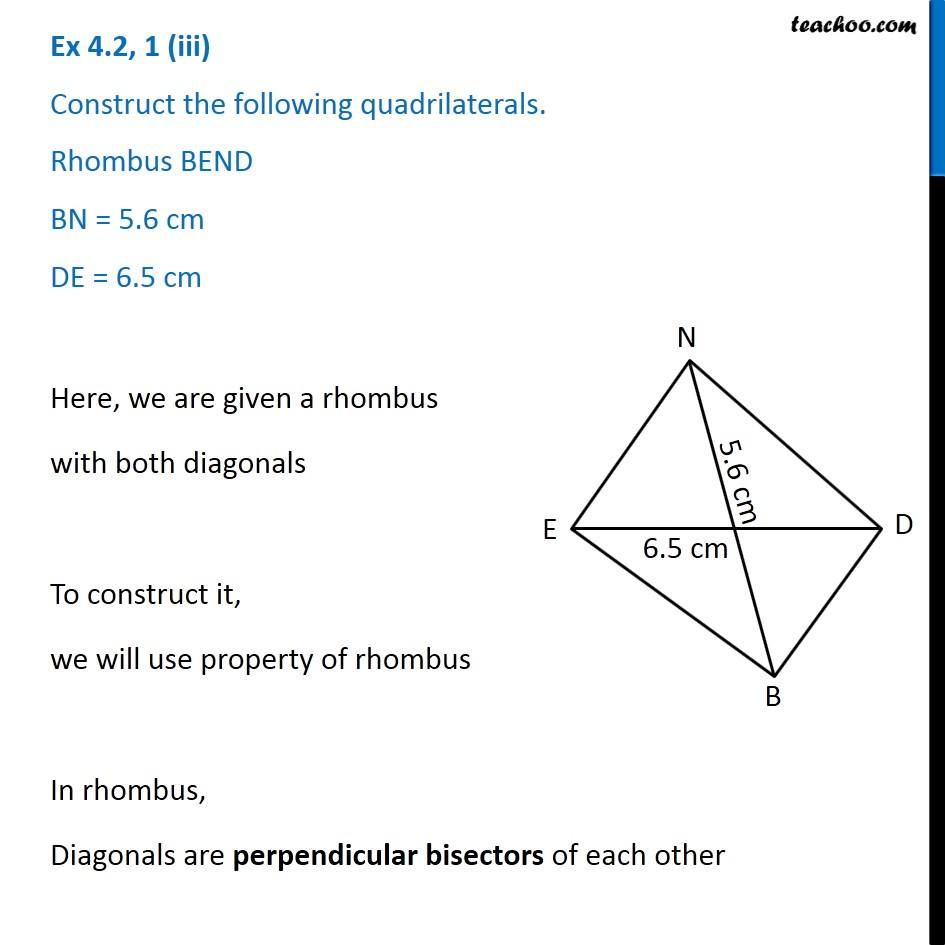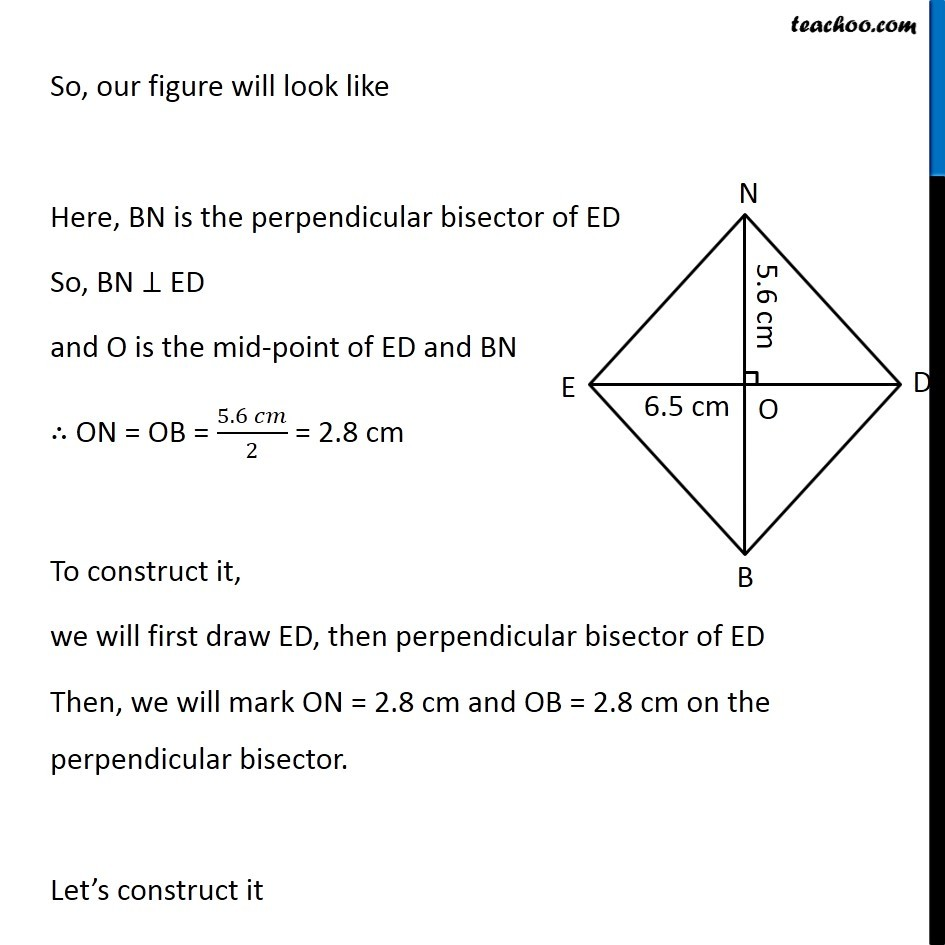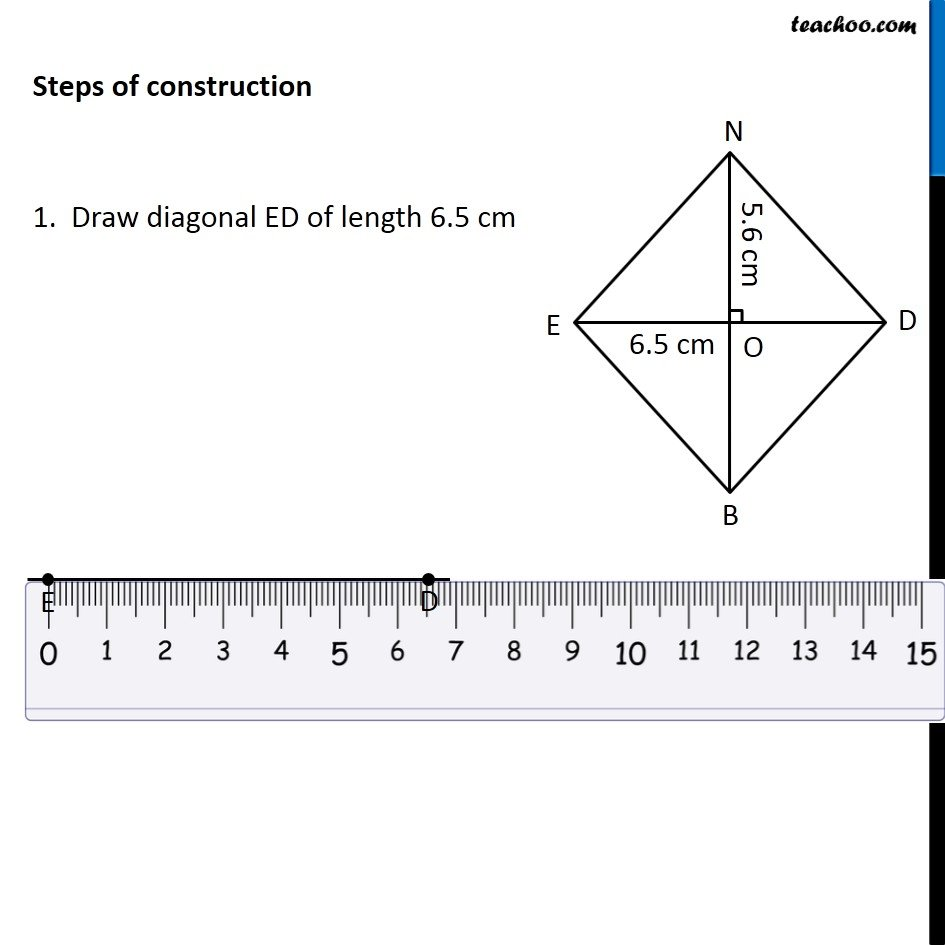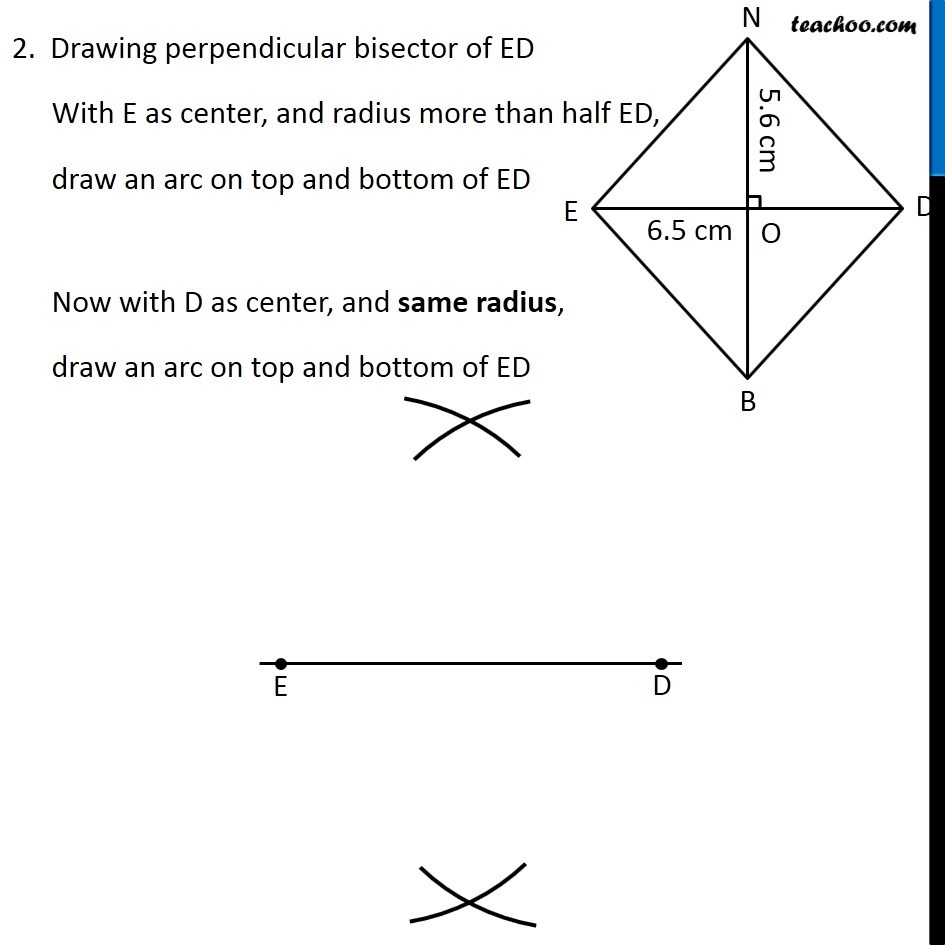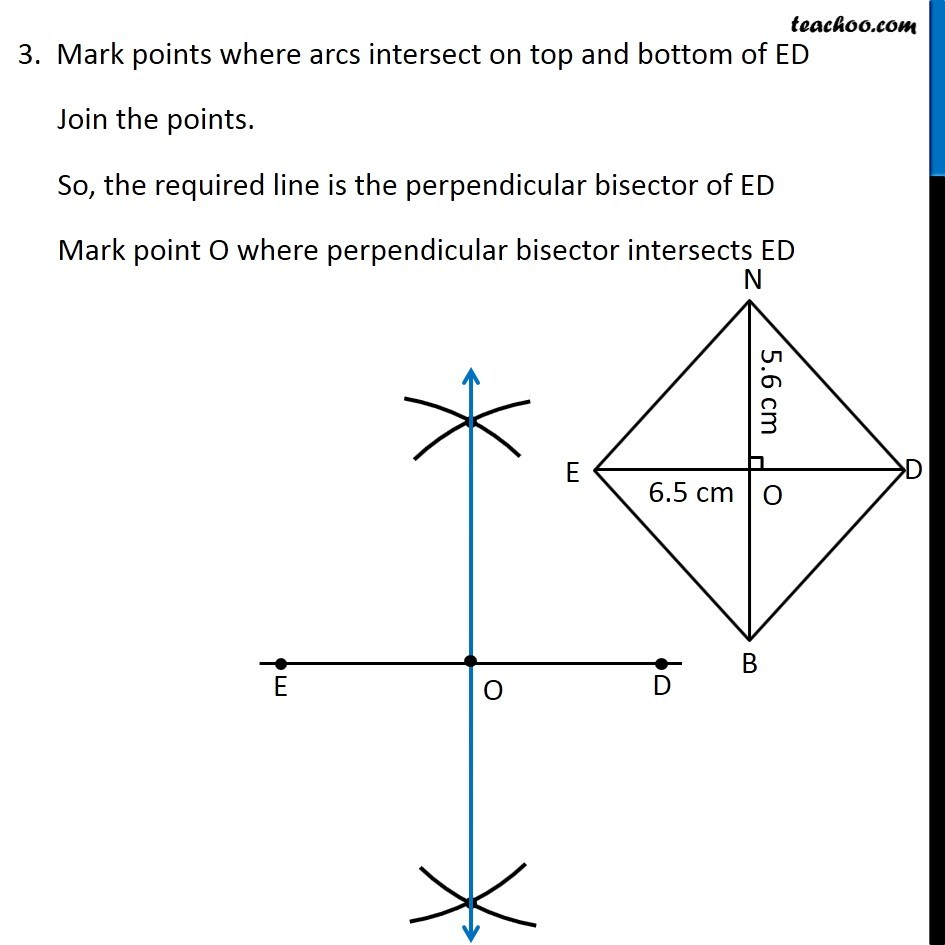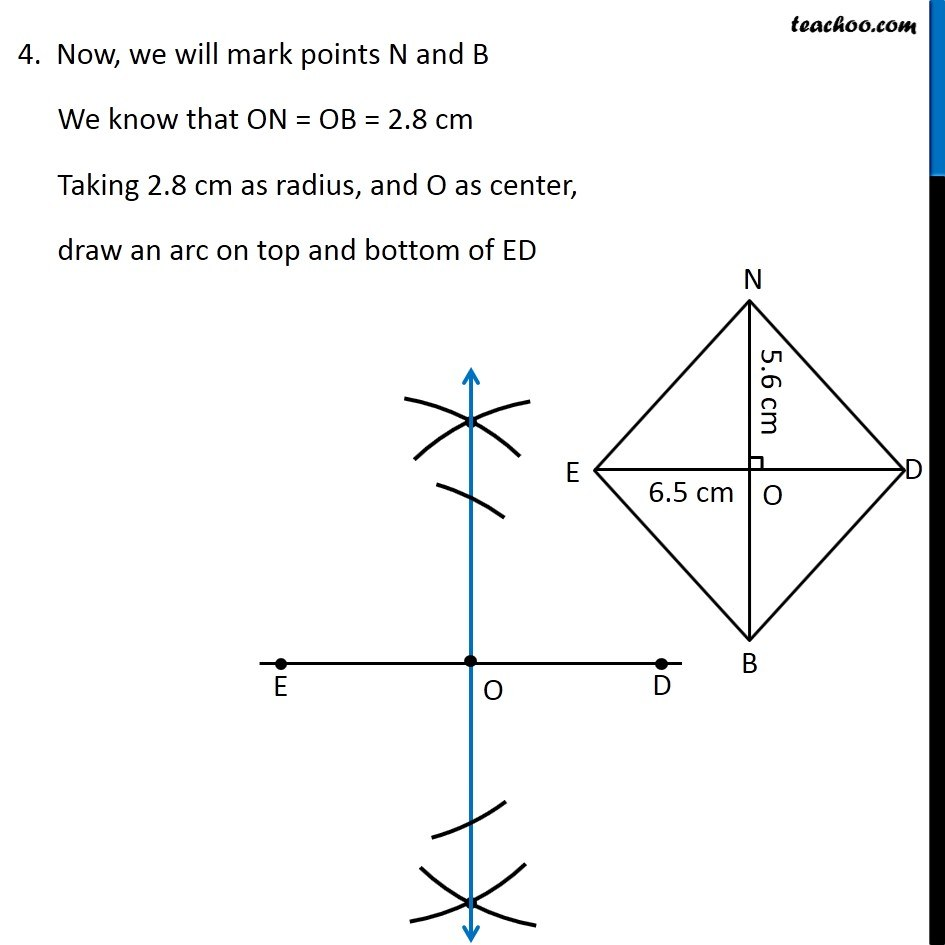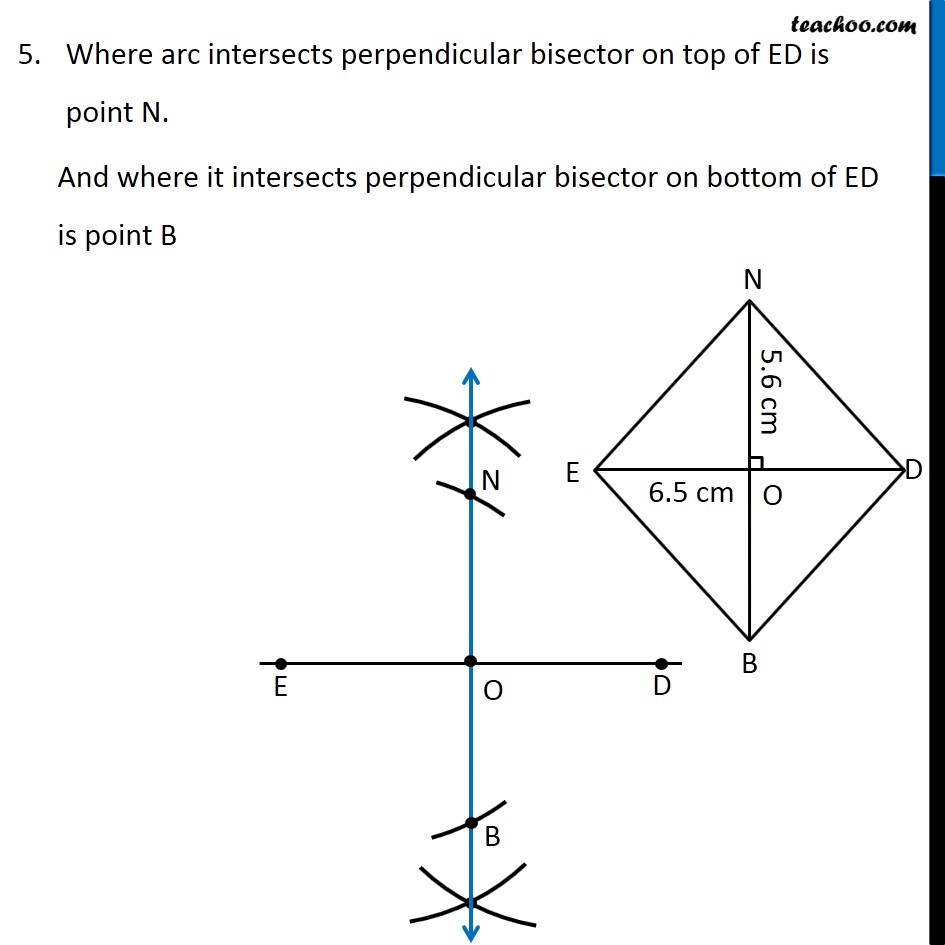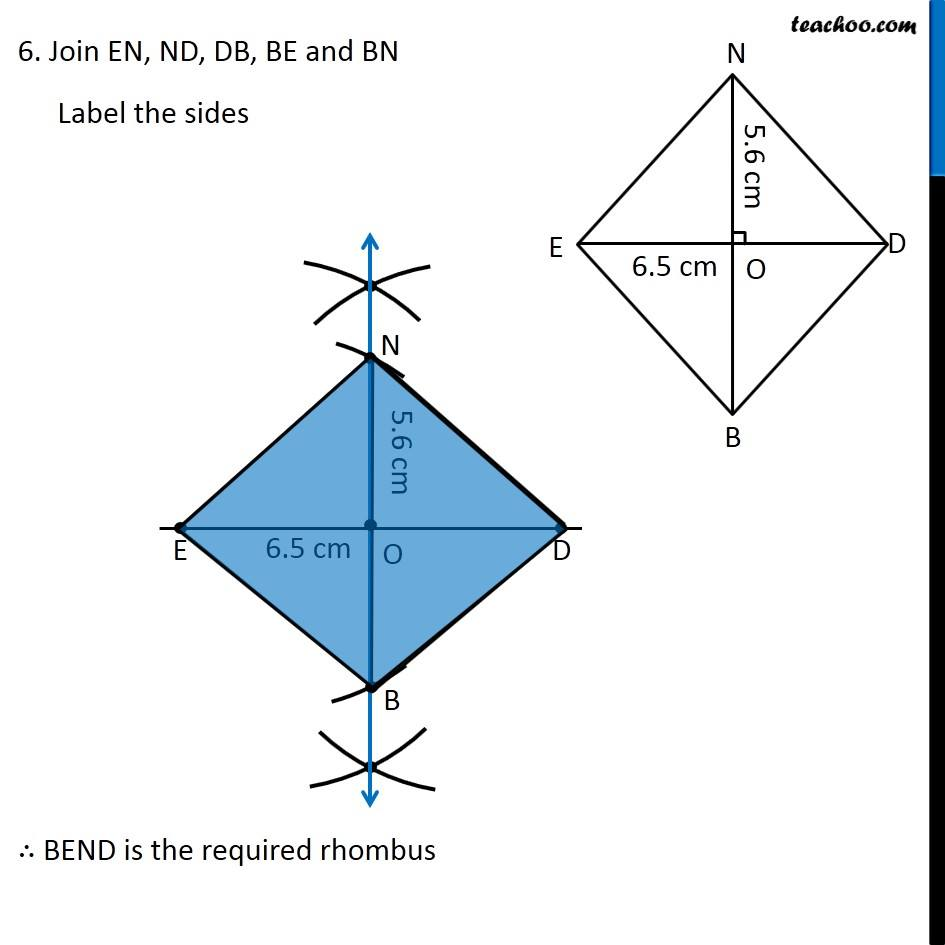Learn in your speed, with individual attention - Teachoo Maths 1-on-1 Class

### Transcript

Question 1 (iii) Construct the following quadrilaterals. Rhombus BEND BN = 5.6 cm DE = 6.5 cm Here, we are given a rhombus with both diagonals To construct it, we will use property of rhombus In rhombus, Diagonals are perpendicular bisectors of each other So, our figure will look like Here, BN is the perpendicular bisector of ED So, BN ⊥ ED and O is the mid-point of ED and BN ∴ ON = OB = (5.6 𝑐𝑚)/2 = 2.8 cm To construct it, we will first draw ED, then perpendicular bisector of ED Then, we will mark ON = 2.8 cm and OB = 2.8 cm on the perpendicular bisector. Let’s construct it Steps of construction 1. Draw diagonal ED of length 6.5 cm 2. Drawing perpendicular bisector of ED With E as center, and radius more than half ED, draw an arc on top and bottom of ED Now with D as center, and same radius, draw an arc on top and bottom of ED 3. Mark points where arcs intersect on top and bottom of ED Join the points. So, the required line is the perpendicular bisector of ED Mark point O where perpendicular bisector intersects ED 4. Now, we will mark points N and B We know that ON = OB = 2.8 cm Taking 2.8 cm as radius, and O as center, draw an arc on top and bottom of ED Where arc intersects perpendicular bisector on top of ED is point N. And where it intersects perpendicular bisector on bottom of ED is point B 6. Join EN, ND, DB, BE and BN Label the sides ∴ BEND is the required rhombus